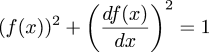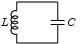# The Universe of Discourse

Sun, 09 Dec 2007

Four ways to solve a nonlinear differential equation
In a recent article I mentioned the differential equation:which I was trying to solve by various methods. The article was actually about calculating square roots of power series; I got sidetracked on this. Before I got back to the original equation, twofour readers of this blog had written in with solutions, all different.

I got interested in this a few weeks ago when I was sitting in on a freshman physics lecture at Penn. I took pretty much the same class when I was a freshman, but I've never felt like I really understood physics. Sitting in freshman physics class again confirms this. Every time I go to a class, I come out with bigger questions than I went in.The instructor was talking about LC circuits, which are simple circuits with a capacitor (that's the "C") and an inductor (that's the "L", although I don't know why). The physics people claim that in such a circuit the capacitor charges up, and then discharges again, repeatedly. When one plate of the capacitor is full of electrons, the electrons want to come out, and equalize the charge on the plates, and so they make a current flowing from the negative to the positive plate. Without the inductor, the current would fall off exponentially, as the charge on the plates equalized. Eventually the two plates would be equally charged and nothing more would happen.

But the inductor generates an electromotive force that tends to resist any change in the current through it, so the decreasing current in the inductor creates a force that tends to keep the electrons moving anyway, and this means that the (formerly) positive plate of the capacitor gets extra electrons stuffed into it. As the charge on this plate becomes increasingly negative, it tends to oppose the incoming current even more, and the current does eventually come to a halt. But by that time a whole lot of electrons have moved from the negative to the positive plate, so that the positive plate has become negative and the negative plate positive. Then the electrons come out of the newly-negative plate and the whole thing starts over again in reverse.

In practice, of course, all the components offer some resistance to the current, so some of the energy is dissipated as heat, and eventually the electrons stop moving back and forth.

Anyway, the current is nothing more nor less than the motion of the electrons, and so it is proportional to the derivative of the charge in the capacitor. Because to say that current is flowing is exactly the same as saying that the charge in the capacitor is changing. And the magnetic flux in the inductor is proportional to rate of change of the current flowing through it, by Maxwell's laws or something.

The amount of energy in the whole system is the sum of the energy stored in the capacitor and the energy stored in the magnetic field of the inductor. The former turns out to be proportional to the square of the charge in the capacitor, and the latter to the square of the current. The law of conservation of energy says that this sum must be constant. Letting f(t) be the charge at time t, then df/dt is the current, and (adopting suitable units) one has:

$$(f(x))^2 + \left(df(x)\over dx\right)^2 = 1$$

which is the equation I was considering.

Anyway, the reason for this article is mainly that I wanted to talk about the different methods of solution, which were all quite different from each other. Michael Lugo went ahead with the power series approach I was using. Say that:

\halign{\hfil \displaystyle #&\displaystyle= #\hfil\cr f & \sum_{i=0}^\infty a_{i}x^{i} \cr f' & \sum_{i=0}^\infty (i+1)a_{i+1}x^{i} \cr }

Then:

\halign{\hfil \displaystyle #&\displaystyle= #\hfil\cr f^2 & \sum_{i=0}^\infty \sum_{j=0}^{i} a_{i-j} a_j x^{i} \cr (f')^2 & \sum_{i=0}^\infty \sum_{j=0}^{i} (i-j+1)a_{i-j+1}(j+1)a_{j+1} x^{i} \cr }

And we want the sum of these two to be equal to 1.

Equating coefficients on both sides of the equation gives us the following equations:

 !!a_0^2 + a_1^2!! = 1 !!2a_0a_1 + 4a_1a_2!! = 0 !!2a_0a_2 + a_1^2 + 6a_1a_3 + 4a_2^2!! = 0 !!2a_0a_3 + 2a_1a_2 + 8a_1a_4 + 12a_2a_3!! = 0 !!2a_0a_4 + 2a_1a_3 + a_2^2 + 10a_1a_5 + 16a_2a_4 + 9a_3^2!! = 0 ...
Now here's the thing M. Lugo noticed that I didn't. You can separate the terms involving even subscripts from those involving odd subscripts. Suppose that a0 and a1 are both nonzero. The polynomial from the second line of the table, 2a0a1 + 4a1a2, factors as 2a1(a0 + 2a2), and one of these factors must be zero, so we immediately have a2 = -a0/2.

Now take the next line from the table, 2a0a2 + a12 + 6a1a3 + 4a22. This can be separated into the form 2a2(a0 + 2a2) + a1(a1 + 6a3). The left-hand term is zero, by the previous paragraph, and since the whole thing equals zero, we have a3 = -a1/6.

Continuing in this way, we can conclude that a0 = -2!a2 = 4!a4 = -6!a6 = ..., and that a1 = -3!a3 = 5!a5 = ... . These should look familiar from first-year calculus, and together they imply that f(x) = a0 cos(x) + a1 sin(x), where (according to the first line of the table) a02 + a12 = 1. And that is the complete solution of the equation, except for the case we omitted, when either a0 or a1 is zero; these give the trivial solutions f(x) = ±1.

Okay, that was a lot of algebra grinding, and if you're not as clever as M. Lugo, you might not notice that the even terms of the series depend only on a0 and the odd terms only on a1; I didn't. I thought they were all mixed together, which is why I alluded to "a bunch of not-so-obvious solutions" in the earlier article. Is there a simpler way to get the answer?

Gareth McCaughan wrote to me to point out a really clever trick that solves the equation right off. Take the derivative of both sides of the equation; you immediately get 2ff' + 2f'f'' = 0, or, factoring out f', f'(f + f'') = 0. So there are two solutions: either f'=0 and f is a constant function, or f + f'' = 0, which even the electrical engineers know how to solve.

David Speyer showed a third solution that seems midway between the two in the amount of clever trickery required. He rewrote the equation as:

$${df\over dx} = \sqrt{1 - f^2}$$

$${df\over\sqrt{1 - f^2} } = dx$$

The left side is an old standby of calculus I classes; it's the derivative of the arcsine function. On integrating both sides, we have:

$$\arcsin f = x + C$$

so f = sin(x + C). This is equivalent to the a0 cos(x) + a1 sin(x) form that we got before, by an application of the sum-of-angles formula for the sine function. I think M. McCaughan's solution is slicker, but M. Speyer's is the only one that I feel like I should have noticed myself.

Finally, Walt Mankowski wrote to tell me that he had put the question to Maple, which disgorged the following solution after a few seconds:

  f(x) = 1, f(x) = -1, f(x) = sin(x - _C1), f(x) = -sin(x - _C1).

This is correct, except that the appearance of both sin(x + C) and -sin(x + C) is a bit odd, since -sin(x + C) = sin(x + (C + π)). It seems that Maple wasn't clever enough to notice that. Walt says he will ask around and see if he can find someone who knows what Maple did to get the solution here.

I would like to add a pithy and insightful conclusion to this article, but I've been working on it for more than a week now, and also it's almost lunch time, so I think I'll have to settle for observing that sometimes there are a lot of ways to solve math problems.